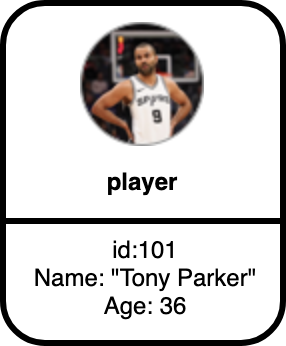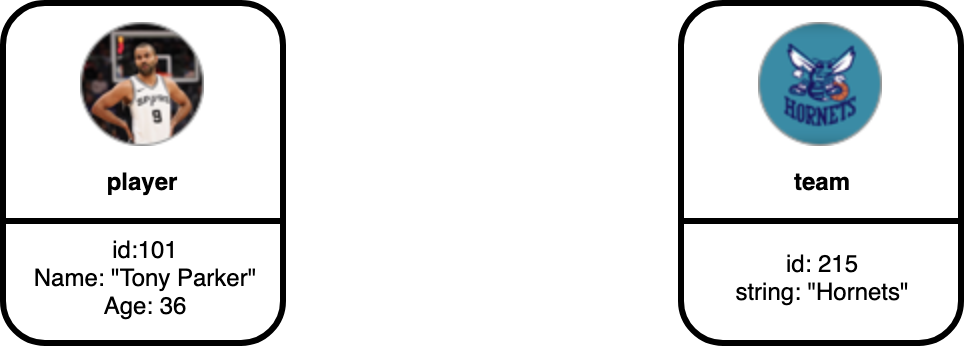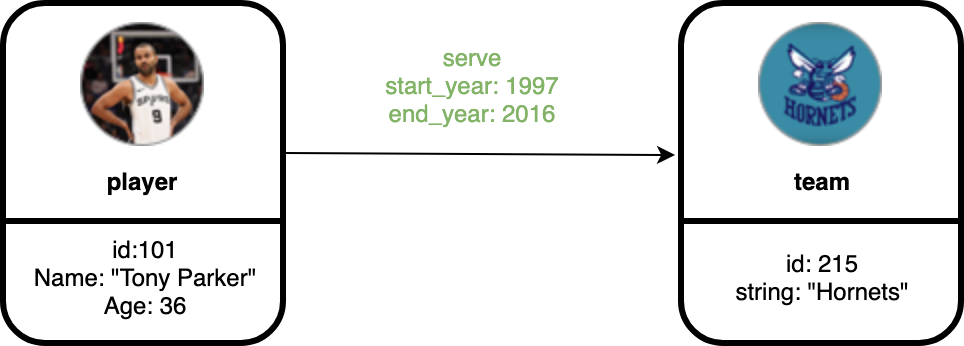# Graph Data Modeling¶

This guide is designed to walk you through the graph data modeling of Nebula Graph. Basic concepts of designing a graph data model will be introduced.

## Graph Space¶

Graph Space is a physically isolated space for different graph. It is similar to database in MySQL.

## Directed Property Graph¶

Nebula Graph stores data in directed property graphs. A directed property graph is a graph of vertices and edges that have a direction. It can be described as follows:

G = < V, E, PV, PE >

• V is the collection of vertices.
• E is the collection of edges.
• PV represents vertex properties.
• PE represents edge properties.

The following table describes a dataset `basketballplayer`. It has two types of vertices, i.e., player and team, and two types of edges, i.e., serve and follow.

Type Name Property (data type) Description
tag player name （string）
age （int）
Represents players.
tag team name （string） Represents teams.
edge type serve start_year （int）
end_year （int）
Represents the behavior of players that connects players with teams.
The direction of the serve edges is from players to teams.
edge type follow degree （int） Represents the behavior of players that connects players.
The direction of the follow edges is from one player to another player.

## Vertices¶

Vertices are typically used to represent entities in the real world. In Nebula Graph, vertices are identified with vertex identifiers (i.e. VIDs). The `VID` must be unique in the graph space. In the preceding example, the graph contains eleven vertices.## Tags¶

In Nebula Graph, vertex properties are clustered by tags. One vertex can have one or more tags. In the preceding example, the vertices have tags player and team.## Edge¶

Edges are used to connect vertices. Each edge usually represents a relationship or a behavior between two vertices. In the preceding example, edges are serve and follow.## Edge Type¶

`Each edge` is an instance of an edge type. Our example uses serve and follow as edge types. Take edge serve for example, in the preceding picture, vertex `101` (represents a player) is the source vertex and vertex `215` (represents a team) is the target vertex. We see that vertex `101` has an outgoing edge while vertex `215` has an incoming edge.

## Properties of Vertices and Edges¶

Both vertices and edges can have properties. Properties are described with key value pairs. In our example graph, we have used the properties `id`, `name` and `age` on player, `id` and `name` on team, and `degree` on follow edge.

## Edge Rank¶

Edge rank is an immutable user-assigned 64-bit signed integer. It affects the edge order of the same edge type between two vertices. The edge with a higher rank value comes first. When not specified, the default rank value is zero. The current sorting basis is "binary coding order", i.e. 0, 1, 2, ... 9223372036854775807, -9223372036854775808, -9223372036854775807, ..., -1. In addition to an edge type, the edge between two vertices must have an edge rank. The edge rank is a 64-bit integer assigned by the user; if not specified, the edge rank defaults to 0.

An edge can be represented uniquely with the [source vertex, edge type, edge rank, destination vertex].

The edge rank affects the edge order of the same edge type between two vertices. The edge with a higher rank value comes first.

The current sorting basis is "binary coding order", i.e. 0, 1, 2, ... 9223372036854775807, -9223372036854775808, -9223372036854775807, ..., -1.

## Schema¶

In Nebula Graph, schema refers to the definition of properties (name, type, etc.). Like `MySQL`, Nebula Graph is a strong typed database. The name and data type of the properties should be determined before the data is written.

Last update: April 16, 2021